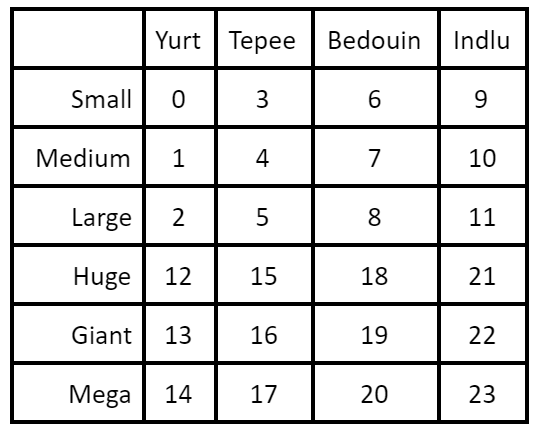Have a question about this project? Sign up for a free GitHub account to open an issue and contact its maintainers and the community.

By clicking “Sign up for GitHub”, you agree to our terms of service and privacy statement. We’ll occasionally send you account related emails.

# What to do if you lose your tent #11

Open
opened this issue Jul 31, 2019 · 0 comments

Owner

### skyjay1 commented Jul 31, 2019

 If you have lost your tent item or destroyed part of the tent in-world, there is still hope! As of Nomadic Tents 9.1.2, you can click on a tent door with an item to obtain a new tent. You can use any item as long as it has the NBT tag "TentCopyTool" set to true. Then, a Creative-mode player uses the item on the door (found in the Tent dimension) and a corresponding tent item is given to the player. The following is an example of how to obtain a stick that will function as a Tent Copy Tool. Simply click on a tent door while holding this item and you will receive a copy of the tent item with the correct NBT information. /give @p stick 1 0 {TentCopyTool:true} If using the tent copy tool is not an option (for example, the door is destroyed in the tent dimension as well as the overworld), you will need to /give yourself an Itemstack with very specific NBT information. For 9.4.x+ use the following template: /give @p yurtmod:tent 1 0 {TentData:{TentType:A,WidthCur:B,DepthCur:C,WidthPrev:D,DepthPrev:E,ID:F}} A, B, C, D, E, and F are number values as explained below: A = the type of tent where YURT = 0, TEPEE = 1, BEDOUIN = 2, INDLU = 3 B = the size of the tent where SMALL = 0, MEDIUM = 1, LARGE = 2, HUGE = 3, GIANT = 4, MEGA = 5 C = the number of extra dirt layers where 0 = NON-UPGRADED and maximum value is 5 = FULLY UPGRADED D = same as B, usually E = same as C, usually F = the location ID of the tent. Starts at 1 and increments for each tent built. Using the value -32768 will cause the tent to dynamically find the next available space. If the X and Z coordinates of the door are known, the ID is given by the formula ID = (X / 32) + (Z / 32) * 64 Notes for 9.5.0+ The tags "WidthPrev" and "DepthPrev" are no longer used, they can be omitted. Also, Shamiyana tents contain a tag "TentColor" which is defined by an integer between 0 and 15. This tag is not required and will default to White when left unspecified. ------------------------------------------------------------------------------------------------- For 9.1.x through 9.3.x, use the following template: /give @p yurtmod:tent 1 0 {TentData:{ StructureTentType:A, StructureWidthCurrent:B, StructureDepthCurrent:C, StructureWidthPrevious:D, StructureDepthPrevious:E, StructureOffsetX:F,< StructureOffsetZ:G}} A, B, C, D, E, F and G are number values as explained below: A = the type of tent where YURT = 0, TEPEE = 1, BEDOUIN = 2, INDLU = 3 B = the size of the tent where SMALL = 0, MEDIUM = 1, LARGE = 2, HUGE = 3, GIANT = 4, MEGA = 5 C = the number of extra dirt layers where 0 = NON-UPGRADED and maximum value is 5 = FULLY UPGRADED D = same as B, usually E = same as C, usually F = the total number of tents of this type (see A) that have been built. If this is the first tent of its type, value is 1. If you know the x position of the tent DOOR in the Tent Dimension, divide that number by 32. G = same as A, usually ----------------------------------------------------------------------------------------- For versions prior to 9.1.2: Admins can use /give to get a new tent item (which will lead to the same tent) by using item damage and NBT tags like so (where A, B, and C are integer values that are explained below) : /give @p yurtmod:tent 1 [A] {TentOffsetX:[B],TentOffsetZ:[C]} [A] = the tent type. Values are as follows:[B] = the X offset of the tent. Values are 1 to 32767 and is incremented for each tent that is made. Unfortunately, the only way to know this is to check the X offset of a tent of the same type that was made right after the now-lost tent. Using an NBT explorer tool, you can also examine the world save data for "craftCountXX" where XX is the tent type. [C] = the Z offset of the tent. If the tent has been upgraded (which it usually was), then C is value on the above chart for SMALL size of the tent.

### Repository owner locked and limited conversation to collaborators Jul 31, 2019

to subscribe to this conversation on GitHub. Already have an account? Sign in.
Projects
None yet
You can’t perform that action at this time.